# ML Aggarwal Solutions for Class 6 Maths Chapter 8 Ratio and Proportion

ML Aggarwal Solutions for Class 6 Maths Chapter 8 Ratio and Proportions are provided here to boost the exam preparations and help students score high marks. This Chapter mainly deals with problems based on Ratio and Proportions. The concepts are explained in detail and easy to understand language. The solutions are designed by subject matter experts who have vast academic experience. It is recommended that students refer to the ML Aggarwal Solutions periodically to improve their problem solving skills. Further, this resource helps in boosting their confidence level to achieve high marks in their exams.

Chapter 8 Ratio and Proportion contains answers to the questions of every topic present in this Chapter. Students can now access and easily download the PDF of ML Aggarwal Class 6 Solutions from the links given below.

## Download the PDF of ML Aggarwal Solutions for Class 6 Maths Chapter 8 Ratio and Proportion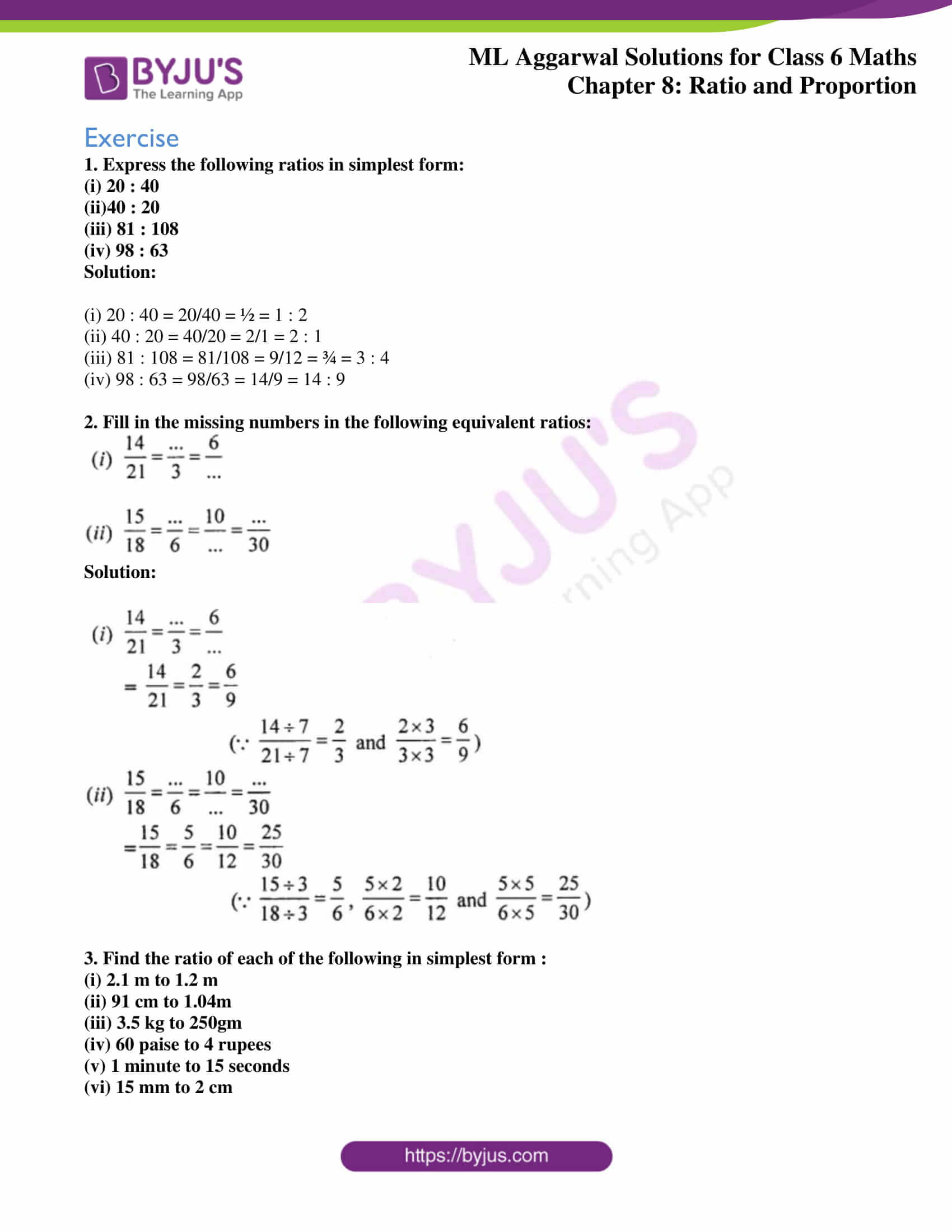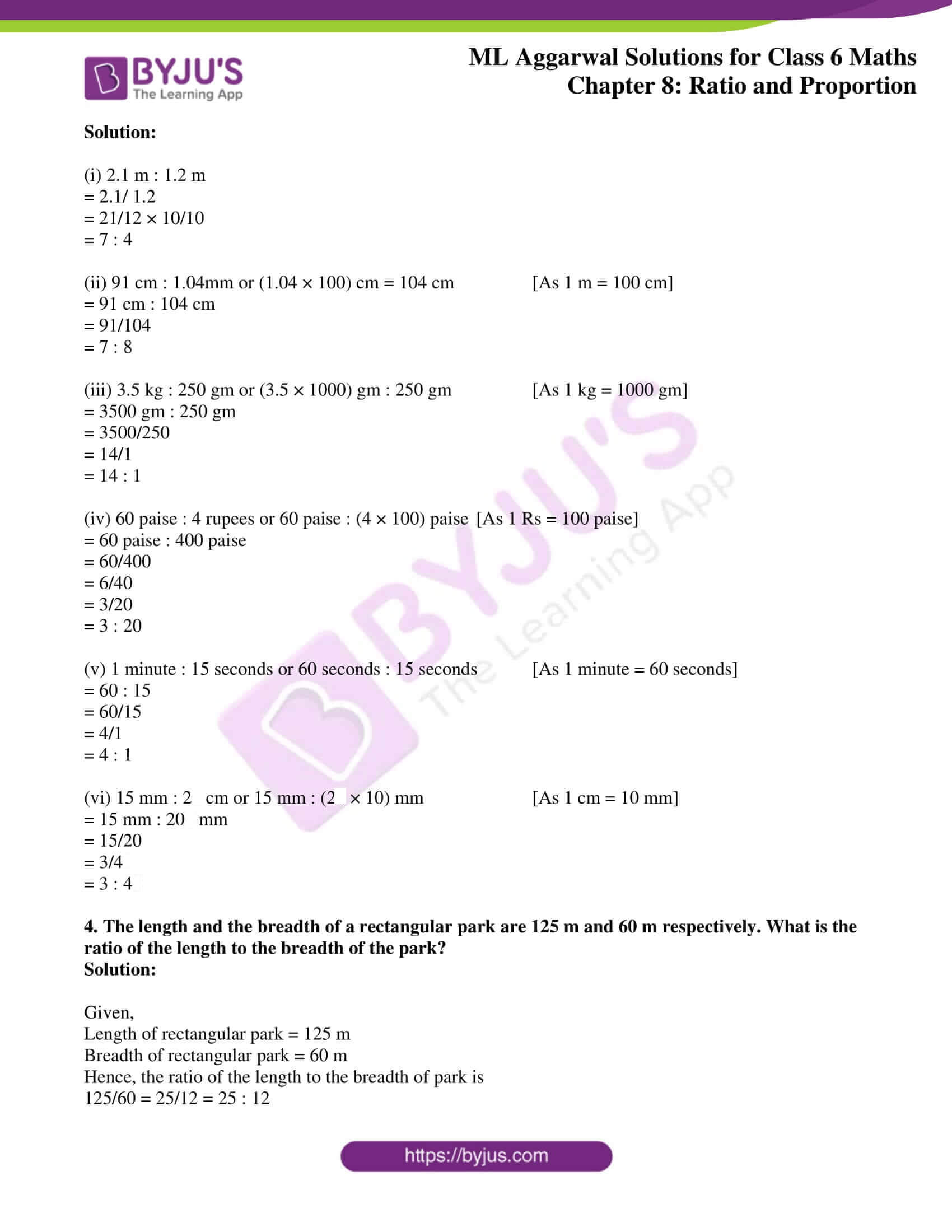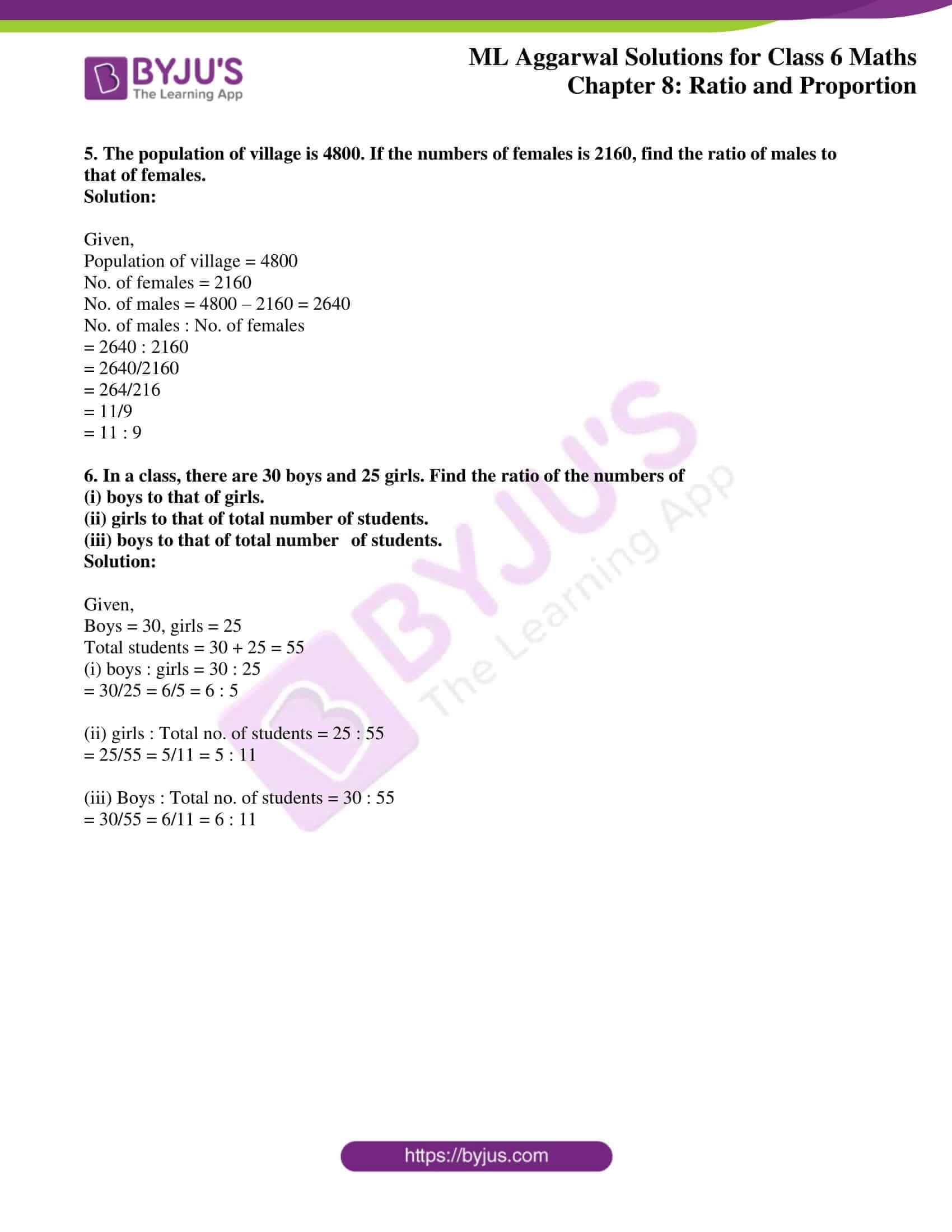### Access answers to ML Aggarwal Solutions for Class 6 Maths Chapter 8 Ratio and Proportion

Exercise
1. Express the following ratios in simplest form:
(i) 20 : 40
(ii)40 : 20
(iii) 81 : 108
(iv) 98 : 63
Solution:

(i) 20 : 40 = 20/40 = ½ = 1 : 2

(ii) 40 : 20 = 40/20 = 2/1 = 2 : 1

(iii) 81 : 108 = 81/108 = 9/12 = ¾ = 3 : 4

(iv) 98 : 63 = 98/63 = 14/9 = 14 : 9

2. Fill in the missing numbers in the following equivalent ratios::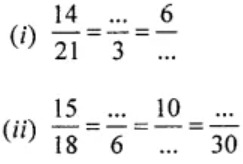Solution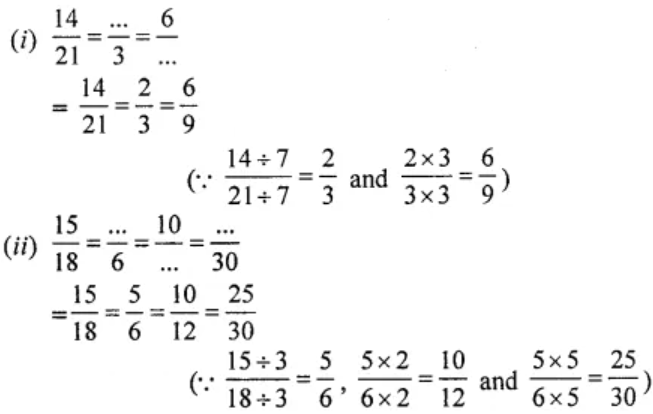3. Find the ratio of each of the following in simplest form :
(i) 2.1 m to 1.2 m
(ii) 91 cm to 1.04m
(iii) 3.5 kg to 250gm
(iv) 60 paise to 4 rupees
(v) 1 minute to 15 seconds
(vi) 15 mm to 2 cm
Solution:

(i) 2.1 m : 1.2 m

= 2.1/ 1.2

= 21/12 × 10/10

= 7 : 4

(ii) 91 cm : 1.04mm or (1.04 × 100) cm = 104 cm [As 1 m = 100 cm]

= 91 cm : 104 cm

= 91/104

= 7 : 8

(iii) 3.5 kg : 250 gm or (3.5 × 1000) gm : 250 gm [As 1 kg = 1000 gm]

= 3500 gm : 250 gm

= 3500/250

= 14/1

= 14 : 1

(iv) 60 paise : 4 rupees or 60 paise : (4 × 100) paise [As 1 Rs = 100 paise]

= 60 paise : 400 paise

= 60/400

= 6/40

= 3/20

= 3 : 20

(v) 1 minute : 15 seconds or 60 seconds : 15 seconds [As 1 minute = 60 seconds]

= 60 : 15

= 60/15

= 4/1

= 4 : 1

(vi) 15 mm : 2 cm or 15 mm : (2 × 10) mm [As 1 cm = 10 mm]

= 15 mm : 20 mm

= 15/20

= 3/4

= 3 : 4

4. The length and the breadth of a rectangular park are 125 m and 60 m respectively. What is the ratio of the length to the breadth of the park?
Solution:

Given,

Length of rectangular park = 125 m

Breadth of rectangular park = 60 m

Hence, the ratio of the length to the breadth of park is

125/60 = 25/12 = 25 : 12

5. The population of village is 4800. If the numbers of females is 2160, find the ratio of males to that of females.
Solution:

Given,

Population of village = 4800

No. of females = 2160

No. of males = 4800 – 2160 = 2640

No. of males : No. of females

= 2640 : 2160

= 2640/2160

= 264/216

= 11/9

= 11 : 9

6. In a class, there are 30 boys and 25 girls. Find the ratio of the numbers of
(i) boys to that of girls.
(ii) girls to that of total number of students.
(iii) boys to that of total number of students.
Solution:

Given,

Boys = 30, girls = 25

Total students = 30 + 25 = 55

(i) boys : girls = 30 : 25

= 30/25 = 6/5 = 6 : 5

(ii) girls : Total no. of students = 25 : 55

= 25/55 = 5/11 = 5 : 11

(iii) Boys : Total no. of students = 30 : 55

= 30/55 = 6/11 = 6 : 11